# Two Dimensional VLOOKUP

The VLOOKUP and HLOOKUP functions are well known for looking up data in one dimension: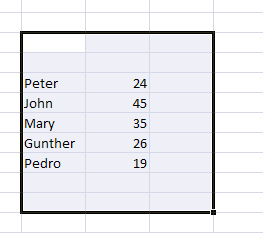And then: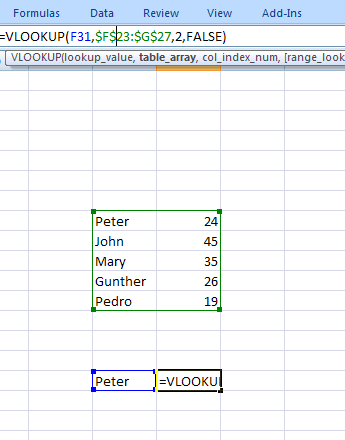However what happens if we have a TWO dimensional array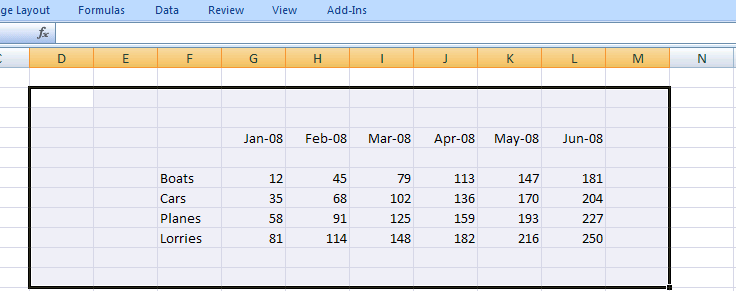And we want to find the value for Boats in Mar-08.  So we could add two drop downs to specify the mode of transport and the month that we need: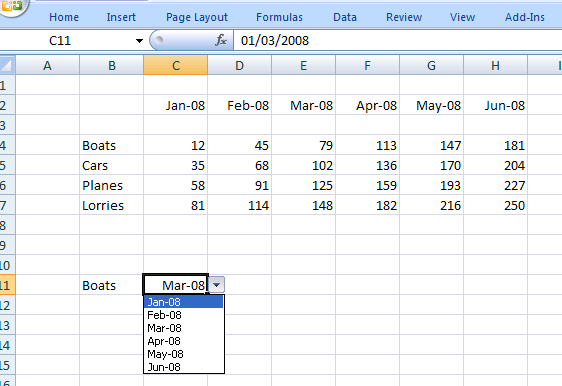Excel provides a function called INDEX that allows us to return values from a 2d array:
INDEX(Array_Range, Row Number, Col Number)

Where
•    Array_Range  is the range in Excel of the two dimensional array – in this case \$B\$4:\$H\$7
•    Row Number  is the position in the list where we find the text “Boat”  – in this case is 1
•    Col Number is the position in the list where we find the month “Mar-08”

Of course the only thing left to do is to determine the Row and Column Number. This is done by using the MATCH function – which returns the position of a string within a range of values:
MATCH(“String”, Range,0) – will return the position of  “String” in the array “Range” and the 0 states that we want an exact match. So we are looking for the position of Boats in the range {Boats, Cars, Planes , Lorries} – which is 1. This will give the row number:

MATCH(B11,\$B\$4:\$B\$7,0)
And similarly for the column number
MATCH(C11,\$C\$2:\$H2,0)
And then we combine all these into one function:
=INDEX(\$C\$4:\$H\$7,MATCH(B11,\$B\$4:\$B\$7,0),MATCH(C11,\$C2:\$H\$2,0))
To give the value of 79 for boats in March 2008: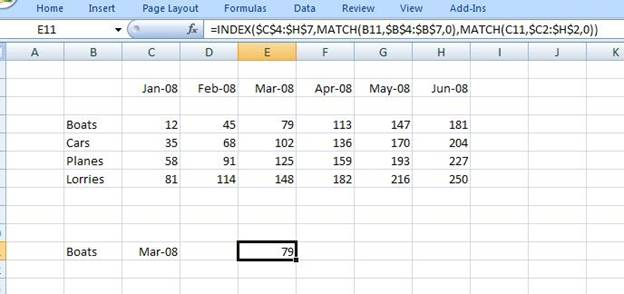### Excel Practice Worksheet

Practice Excel functions and formulas with our 100% free practice worksheets!